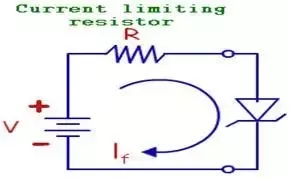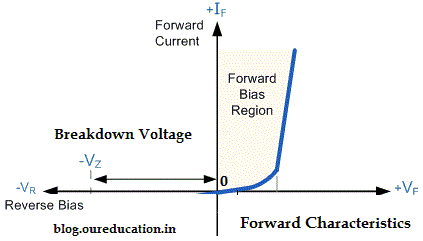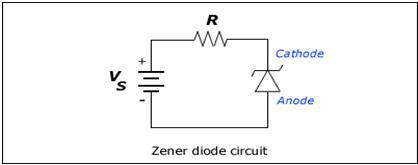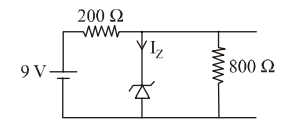### Zener Diode

A Zener diode is a  silicon  semiconductor  device  that  permits  current   to  flow  in either a forward or reverse   direction. The   diode   consists  of a special, heavily  doped p-n junction, designed to  conduct in the reverse direction when a   certain specified voltage is reached.
A Zener diode is a special type of Diode designed to reliably allow current  to flow "backwards" when a certain set reverse voltage,  known as the Zener voltage, is reached.In zener diode there are three node of operation:

1. Forward bias

2. Reverse biased

3. Reverse bias with breakdown

1. Forward Bias:

In forward bias zener diode act as like P N junction diode . in ideal condition it become short circuit with zero v voltage drop And  finite amount of current flowing  through it.   And in practical case   it can be represented with voltage drop and current  through it.1. Reverse Bias:

Zener diode is a heavily doped diode. It acts as a normal diode in forwarding bias. When the Zener diode is reverse biased  the junction potential increases. As the breakdown voltage is high this will provide high voltage handling capacity. In reverse bias it acts like PN junction diode with zero current flowing through it and voltage drop in ideal condition and  practical case small amount of current flows  called minority current due to reverse bias condition.1. Reverse biased with break down:

The Zener diode is connected parallel with  load to make it reverse bias, and once the Zener diode exceeds knee voltage the voltage across the load will become constant. The breakdown voltage of Zener diodes will be constant for  a large  range of currents. When  voltage applied is greater than breakdown voltage then large amount of current flows through it  this condition Called as  zener diode with reverse biased.in above fig when load voltage greater than zener break  down voltage then load voltage becomes constant and  equal to breakdown voltage of diode.

Application of Zener Diode:

1. Zener diode as voltage regulator.

2. As a reference element.

3. Switching applications.

4. Clipper circuit.​​​​​​​